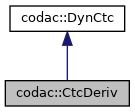codac
codac::CtcDeriv Class Reference

$\mathcal{C}_{\frac{d}{dt}}$ that contracts a tube $[x](\cdot)$ with respect to its derivative tube $[v](\cdot)$ according to the constraint $\dot{x}(\cdot)=v(\cdot)$ More...

#include <codac_CtcDeriv.h>

Inheritance diagram for codac::CtcDeriv:[legend]
Collaboration diagram for codac::CtcDeriv:[legend]

## Public Member Functions

CtcDeriv ()
Creates a contractor object $\mathcal{C}_{\frac{d}{dt}}$.

void contract (Tube &x, const Tube &v, TimePropag t_propa=TimePropag::FORWARD|TimePropag::BACKWARD)
$\mathcal{C}_{\frac{d}{dt}}\big([x](\cdot),[v](\cdot)\big)$: contracts the tube $[x](\cdot)$ with respect to its derivative $[v](\cdot)$. More...

void contract (TubeVector &x, const TubeVector &v, TimePropag t_propa=TimePropag::FORWARD|TimePropag::BACKWARD)
$\mathcal{C}_{\frac{d}{dt}}\big([\mathbf{x}](\cdot),[\mathbf{v}](\cdot)\big)$: contracts the tube $[\mathbf{x}](\cdot)$ with respect to its derivative $[\mathbf{v}](\cdot)$. More...

void contract (Slice &x, const Slice &v, TimePropag t_propa=TimePropag::FORWARD|TimePropag::BACKWARD)
$\mathcal{C}_{\frac{d}{dt}}\big(\llbracket x\rrbracket(\cdot),\llbracket v\rrbracket(\cdot)\big)$: contracts the slice $\llbracket x\rrbracket(\cdot)$ with respect to its derivative $\llbracket v\rrbracket(\cdot)$. More...Public Member Functions inherited from codac::DynCtc
DynCtc (bool intertemporal=true)
Creates a contractor. More...

virtual ~DynCtc ()
DynCtc destructor.

void preserve_slicing (bool preserve=true)
Specifies whether the contractor can impact the tube's slicing or not. More...

void set_fast_mode (bool fast_mode=true)
Specifies an optional fast mode of contraction. More...

void restrict_tdomain (const Interval &tdomain)
Limits the temporal domain of contractions. More...

bool is_intertemporal () const
Tests if the related constraint is inter-temporal or not. More...

## Protected Member Functions

void contract_gates (Slice &x, const Slice &v)
Contracts input and output gates of a slice regarding its derivative set. More...

## Static Protected Attributes

static const std::string m_ctc_name
class name (mainly used for CN Exceptions)

static std::vector< std::string > m_str_expected_doms
allowed domains signatures (mainly used for CN Exceptions)Protected Attributes inherited from codac::DynCtc
bool m_preserve_slicing = true
if true, tube's slicing will not be affected by the contractor

bool m_fast_mode = false
some contractors may propose more pessimistic but faster execution modes

Interval m_restricted_tdomain
limits the contractions to the specified temporal domain

const bool m_intertemporal = true
defines if the related constraint is inter-temporal or not (true by default)

## Detailed Description

$\mathcal{C}_{\frac{d}{dt}}$ that contracts a tube $[x](\cdot)$ with respect to its derivative tube $[v](\cdot)$ according to the constraint $\dot{x}(\cdot)=v(\cdot)$

Note
This contractor is called $\mathcal{C}_{\frac{d}{dt}}$ in the literature.
Todo:
Contract the tube $[v](\cdot)$ if $[x](\cdot)$ degenerated?

## ◆ contract() [1/3]

 void codac::CtcDeriv::contract ( Tube & x, const Tube & v, TimePropag t_propa = TimePropag::FORWARD|TimePropag::BACKWARD )

$\mathcal{C}_{\frac{d}{dt}}\big([x](\cdot),[v](\cdot)\big)$: contracts the tube $[x](\cdot)$ with respect to its derivative $[v](\cdot)$.

Precondition
$[x](\cdot)$ and $[v](\cdot)$ must share the same slicing and tdomain.
Parameters
 x the scalar tube $[x](\cdot)$ v the scalar derivative tube $[v](\cdot)$ t_propa an optional temporal way of propagation (forward or backward in time, both ways by default)

## ◆ contract() [2/3]

 void codac::CtcDeriv::contract ( TubeVector & x, const TubeVector & v, TimePropag t_propa = TimePropag::FORWARD|TimePropag::BACKWARD )

$\mathcal{C}_{\frac{d}{dt}}\big([\mathbf{x}](\cdot),[\mathbf{v}](\cdot)\big)$: contracts the tube $[\mathbf{x}](\cdot)$ with respect to its derivative $[\mathbf{v}](\cdot)$.

Precondition
$[\mathbf{x}](\cdot)$ and $[\mathbf{v}](\cdot)$ must share the same dimension, slicing and tdomain.
Parameters
 x the n-dimensional tube $[\mathbf{x}](\cdot)$ v the n-dimensional derivative tube $[\mathbf{v}](\cdot)$ t_propa an optional temporal way of propagation (forward or backward in time, both ways by default)

## ◆ contract() [3/3]

 void codac::CtcDeriv::contract ( Slice & x, const Slice & v, TimePropag t_propa = TimePropag::FORWARD|TimePropag::BACKWARD )

$\mathcal{C}_{\frac{d}{dt}}\big(\llbracket x\rrbracket(\cdot),\llbracket v\rrbracket(\cdot)\big)$: contracts the slice $\llbracket x\rrbracket(\cdot)$ with respect to its derivative $\llbracket v\rrbracket(\cdot)$.

Precondition
$\llbracket x\rrbracket(\cdot)$ and $\llbracket v\rrbracket(\cdot)$ must share the same tdomain.
Parameters
 x the slice $\llbracket x\rrbracket(\cdot)$ v the derivative slice $\llbracket v\rrbracket(\cdot)$ t_propa an optional temporal way of propagation (forward or backward in time, both ways by default)

## ◆ contract_gates()

 void codac::CtcDeriv::contract_gates ( Slice & x, const Slice & v )
protected

Contracts input and output gates of a slice regarding its derivative set.

Precondition
$\llbracket x\rrbracket(\cdot)$ and $\llbracket v\rrbracket(\cdot)$ must share the same tdomain.
Note
Only the gates may be affected. This method is used for the $\mathcal{C}_\textrm{eval}$ contractor.
Parameters
 x the slice $\llbracket x\rrbracket(\cdot)$ v the derivative slice $\llbracket v\rrbracket(\cdot)$

The documentation for this class was generated from the following file: